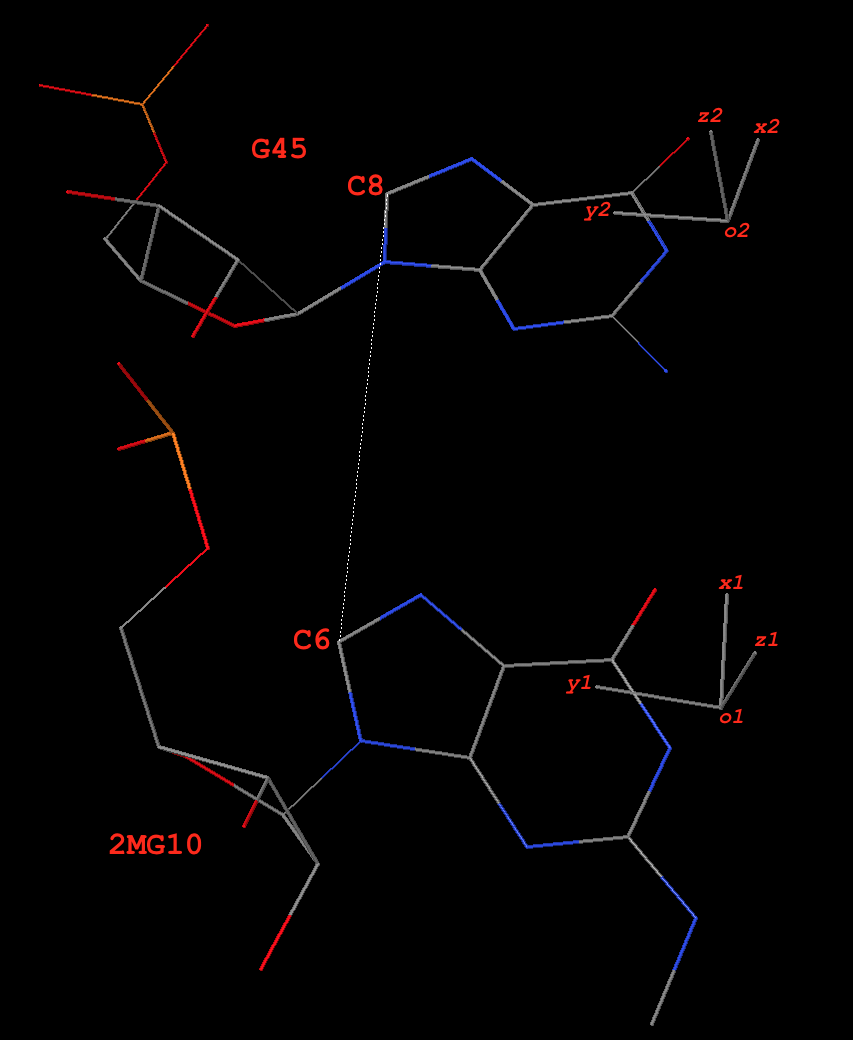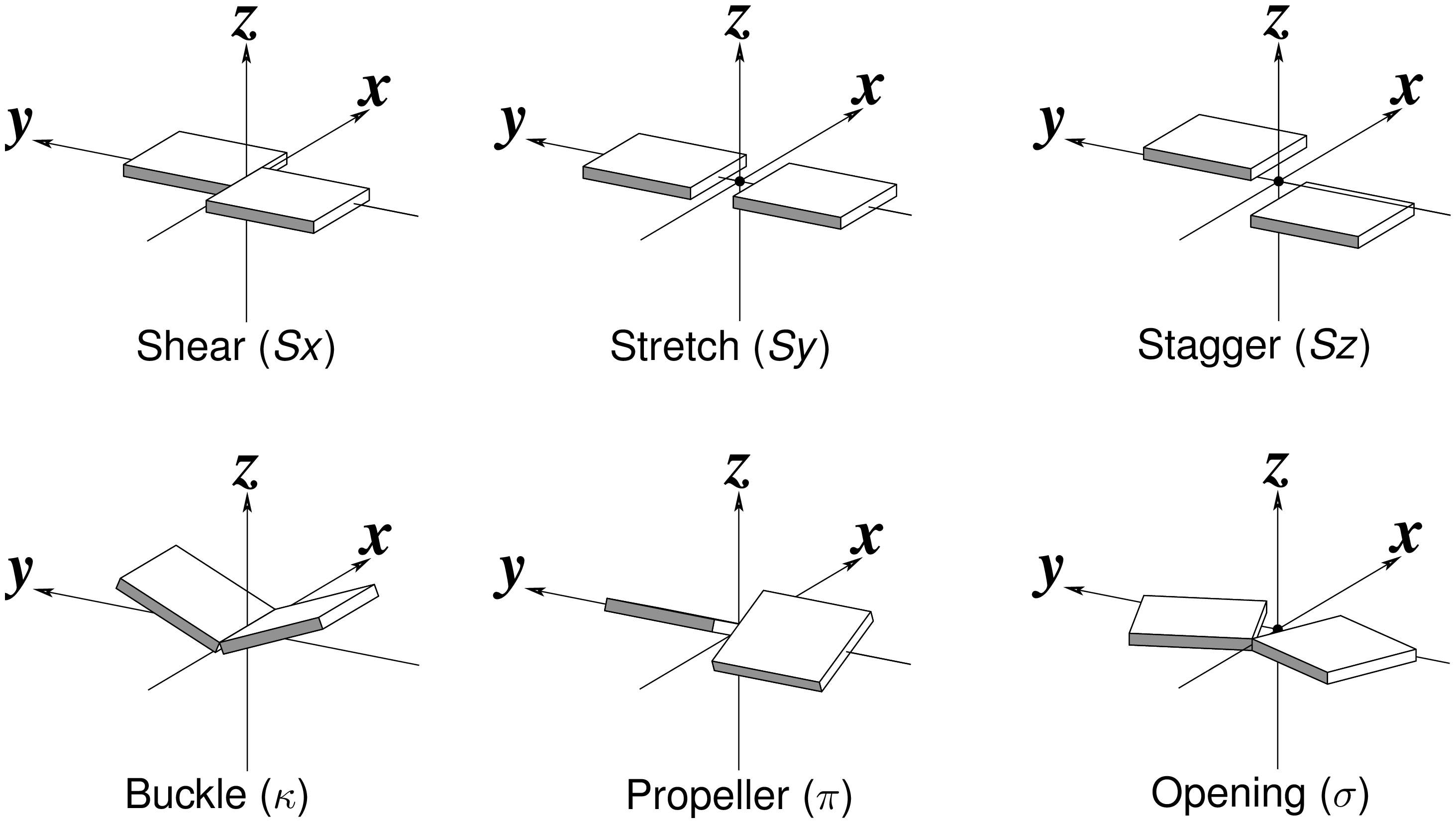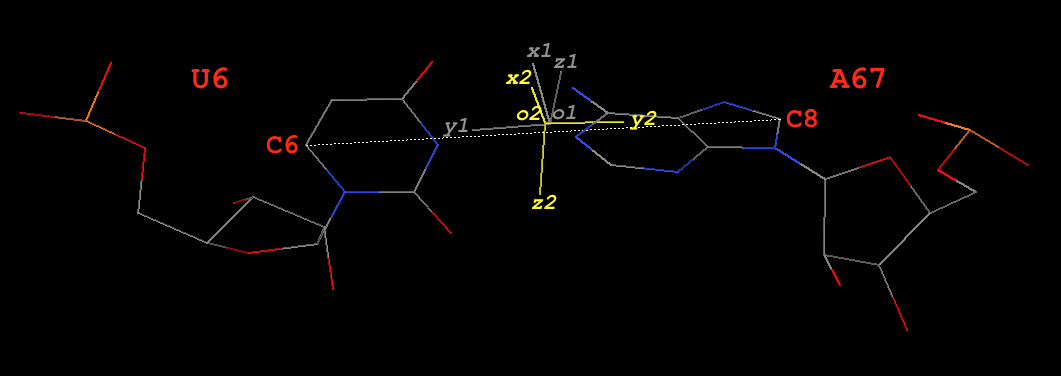# Details on the simple base-pair parameters

With the foundation laid by the previous two posts on Fitting of base reference frame and Automatic identification of nucleotides, we can now get into the details on how the ‘simple’ base-pair (bp) parameters are derived. To make the point clear, I am using two concrete examples from the yeast phenylalanine tRNA (PDB id: 1ehz): the first pair is 2MG10+G45, of type `M+N` (shortened to `g+G`) in 3DNA/DSSR; and the second example is a Watson-Crick pair U6–A67, of type `M–N` (shortened to `U–A`).

# Pair 2MG10+G45 (g+G, of type `M+N`, see Fig. 1)#### Base reference framesFig. 1: Base pair 2MG10+G45 (g+G) of type `M+N` in yeast phenylalanine tRNA 1ehz

In the original coordinate system (as in `1ehz.pdb` downloaded from the RCSB PDB), the base-reference frames for 2MG10 and G45 are:

```# base reference frame of 2MG10
{
"rsmd": 0.018218,
"origin": [65.696016, 45.134944, 18.125044],  # o1
"x_axis": [0.690346, 0.713907, -0.117302],    # x1
"y_axis": [-0.706849, 0.700116, 0.101003],    # y1
"z_axis": [0.154232, 0.013188, 0.987947]      # z1
}
# base reference frame of G45
{
"rsmd": 0.025865,
"origin": [70.584399, 50.526567, 17.229626],  # o2
"x_axis": [0.818521, 0.49914, -0.284399],     # x2
"y_axis": [-0.574112, 0.728382, -0.373973],   # y2
"z_axis": [0.020486, 0.469381, 0.882758]      # z2
}```

#### The base-pair reference frame

Since `dot(z1, z2) = 0.88` (positive), this pair is of type `M+N` in 3DNA/DSSR. The ‘mean’ z-axis of the pair is the average of z1 and z2, which is `z = [0.090069, 0.248769, 0.964366]` (normalized). This is the z-axis of the bp frame, as in 3DNA/DSSR.

The ‘long’ axis employs RC8 (purines) and YC6 (pyrimidines) base atoms. Here 2MG10 and G45 are all purines, so the following two C8 atoms are used:

```# C8 atoms of 2MG10 and G45 in 1ehz
HETATM  208  C8  2MG A  10      62.199  48.621  18.635  1.00 40.38           C
ATOM    987  C8    G A  45      67.772  54.149  15.386  1.00 40.45           C```

The vector from C8 of G45 to C8 of 2MG10 is:

```y0 = [62.199  48.621  18.635] - [67.772  54.149  15.386]
= [-5.573  -5.528   3.249]```

Normally, y0 and z-axis are not orthogonal. Here they have an angle of ~81º. The orthogonal component of y0 with reference to the z-axis, when normalized, is the y-axis:

`y = [-0.676751, -0.695120, 0.242520]`

The x-axis is defined by the right-handed rule:

`x = [-0.730682, 0.674479, -0.105746]`

Overall, the orthonormal x-, y- and z-axes of the pair defined thus far are:

```x = [-0.730682, 0.674479, -0.105746]
y = [-0.676751, -0.695120, 0.242520]
z = [0.090069, 0.248769, 0.964366]```

#### Derivation of the six ‘simple’ base-pair parameters (Fig. 2)Fig. 2: Schematic diagram of six rigid-body base-pair parameters

Propeller is the ‘torsion’ angle of z2 to z1 with reference to the y-axis, and is calculated using the method detailed in the blog post How to calculate torsion angle?. Here Propeller is: `-24.24`º. Similarly, Buckle is defined as the ‘torsion’ angle of z2 to z1 with reference to the x-axis, and is `-14.81`º. Opening is defined as the angle from y2 to y1 with reference to the z-axis, and is: `13.32`º.

The corresponding translational parameters are simply projects of the o2 to o1 vector onto the x-, y- and z-axis, respectively. Here, they have values:

```d = o1 - o2 = [-4.888383, -5.391623, 0.895418]
Shear = dot(d, x) = -0.16
Stretch = dot(d, y) = 7.27
Stagger = dot(d, z) = -0.92```

#### ‘Corrections’ of Buckle and Propeller

Base-pair non-planarity is due to the following three parameters: Buckle, Propeller, and Stagger. In particular, Buckle and Propeller cause the two bases to be non-parallel, the most noticeable characteristic of a pair. These two angular parameters are well-documented in literature, even among the canonical Watson-Crick base pairs. In 3DNA/DSSR, the angle between the two base normal vectors (in range [0, 90º]) is related to Buckle and Propeller with the formula:

`interBase-angle = sqrt(Buckle^2+Propeller^2)`

For the 2MG10+G45 pair, the angle between z1 and z2 is `28.18`º, and `sqrt(Buckle^2+Propeller^2) = 28.405`º. So the following ‘corrections’ are made:

```Buckle = -14.81 * 28.18 / 28.405 = -14.69
Propeller = -24.24 * 28.18 / 28.405 = -24.05```

Overall, the ‘corrections’ have only small influence on the numerical values of the reported Buckle and Propeller parameters. It is ‘sensible’ that the ‘simple’ parameters have the property `interBase-angle = sqrt(Buckle^2+Propeller^2)`, just as the original 3DNA/DSSR bp parameters.

Now, the six ‘simple’ bp parameters for 2MG10+G45, reported in 3DNA `analyze` program as of v2.3-2016jan01 are:

```Simple base-pair parameters based on YC6-RC8 vectors
bp        Shear    Stretch   Stagger    Buckle  Propeller  Opening  angle
*    1 g+G      -0.16      7.27     -0.92    -14.69    -24.05     13.32   28.2```

The corresponding local bp parameters as originally reported by 3DNA/DSSR are as follows. Note the significant differences in Shear vs. Stretch, and Buckle vs. Propeller in the two sets of bp parameters. On the other hand, Stagger is identical and Opening should be quite close, by definition. Due to the similarity in Stagger and Opening, DSSR only reports four ‘simple’ parameters (i.e., Shear, Stretch, Buckle, and Propeller).

```Local base-pair parameters
bp        Shear    Stretch   Stagger    Buckle  Propeller  Opening
1 g+G      -7.21     -0.97     -0.92     25.58    -11.83     13.07```

# Base-pair U6–A67 (Watson-Crick U–A, of type `M–N`, see Fig. 3)Fig. 3: Base pair U6–A67 (U–A) of type `M–N` in yeast phenylalanine tRNA 1ehz

#### Base reference frames

In the original coordinate system (as in `1ehz.pdb` downloaded from the RCSB PDB), the base-reference frames for U6 and A67 are:

```# base reference frame of U6 (white in Fig. 3)
{
"rsmd": 0.010835,
"origin": [60.441988, 48.83479, 41.242523],  # o1
"x_axis": [0.28491, 0.503019, 0.815965],     # x1
"y_axis": [0.887155, -0.460753, -0.025726],  # y1
"z_axis": [0.363018, 0.731217, -0.577529]    # z1
}
# base reference frame of A67 (colored yellow in Fig. 3)
{
"rsmd": 0.01992,
"origin": [60.578326, 48.823104, 41.154211], # o2
"x_axis": [0.034097, 0.205538, 0.978055],    # x2
"y_axis": [-0.90687, 0.417653, -0.056155],   # y2
"z_axis": [-0.420029, -0.885054, 0.200637]   # z2
}```

#### The base-pair reference frame

Since `dot(z1, z2) = -0.92` (negative), this pair is of type `M–N` in 3DNA/DSSR. The y- and z-axis are thus reversed (corresponding to a 180º rotation around the x-axis) to align z2 with z1.

```# base reference frame of A67, with y- and z-axes reversed
{
"origin": [60.578326, 48.823104, 41.154211], # o2
"x_axis": [0.034097, 0.205538, 0.978055],    # x2
"y_axis": [0.90687, -0.417653, 0.056155],    # y2 -- reversed
"z_axis": [0.420029, 0.885054, -0.200637]    # z2 -- reversed
}```

Thereafter, the procedure is similar to the one for the `M+N` type above. Note here U6 is a pyrimidine, so its C6 atom is used. The final results are:

```# C6 atom of U6 and C8 atom A67 in 1ehz
ATOM    132  C6    U A   6      64.926  46.497  41.084  1.00 35.72           C
ATOM   1457  C8    A A  67      56.129  50.866  40.893  1.00 40.04           C
#---------
y0 = [64.926  46.497  41.084] - [56.129  50.866  40.893]
= [8.797  -4.369   0.191]
x = [0.160777, 0.363836, 0.917482]
y = [0.902274, -0.430972, 0.012793]
z = [0.400064, 0.825764, -0.397570]```

#### The six ‘simple’ and original base-pair parameters

```Simple base-pair parameters based on YC6-RC8 vectors
bp        Shear    Stretch   Stagger    Buckle  Propeller  Opening  angle
1 U-A       0.06     -0.13     -0.08     -0.59    -23.71      5.39   23.7
# ------------
Local base-pair parameters
bp        Shear    Stretch   Stagger    Buckle  Propeller  Opening
1 U-A       0.06     -0.13     -0.08     -0.63    -23.71      5.50```

As can be seen, for Watson-Crick pairs, the ‘simple’ and the original bp parameters are very similar.

# Special notes on the ‘simple’ base-pair parameters• For the most common Watson-Crick pairs, the newly introduced ‘simple’ bp parameters match those of the original 3DNA/DSSR parameters very well (as shown by the U6–A67 pair). For non-canonical pairs, significant differences in Shear, Stretch, Buckle and Propeller are expected (as illustrated by the 2MG10+G45 pair). The differences come from the divergent definitions of the bp reference frame, which is distinct for each type of non-canonical pairs.
• Only the original 3DNA/DSSR six bp parameters can be used for exact reconstruction (with the 3DNA `rebuild` program) of the corresponding bp geometry. The ‘simple’ bp parameters are for description only, and they could be more intuitive than the original 3DNA/DSSR counterparts. They complement, buy by no means replace, the classic “local” bp parameters. The term ‘simple’ is used to distinguish the new from the original closely related, yet quite different bp parameters.
• As details for the 2MG10+G45 pair, several ad hoc decisions are made in deriving the ‘simple’ bp parameters. For example, instead of using RC8–YC6 to define the y-axis, one can also use RN9–YN1 (as did by Richardson). Each such choice will lead (slightly) different numerical values, depending on the type of the non-canonical pairs. In some cases, Buckle and Propeller could differ by several degrees. Since RC8 and YC6 atoms lie near the ‘center’ of purines and pyrimidines, they are used to define the y-axis (by default). DSSR has provisions of selecting RN9–YN1, as well as a couple of other choices, for the definition of the y-axis.
• When the M+N pair is counted as N+M, Shear, Stretch, Buckle, and Propeller remain the same, but Stagger and Opening reverse their signs. For example, here are the results of 2MG10+G45 vs. G45+2MG10:
```# 2MG10+G45
Simple base-pair parameters based on YC6-RC8 vectors
bp        Shear    Stretch   Stagger    Buckle  Propeller  Opening  angle
*    1 G+g      -0.16      7.27      0.92    -14.69    -24.05    -13.32   28.2
# Reverse the order: treated as G45+2MG10
Simple base-pair parameters based on YC6-RC8 vectors
bp        Shear    Stretch   Stagger    Buckle  Propeller  Opening  angle
*    1 g+G      -0.16      7.27     -0.92    -14.69    -24.05     13.32   28.2```
• When the M–N pair is counted as N–M, Stretch, Stagger, Propeller, and Opening remain the same, but Shear and Buckle reverse their signs. For example, here are the results of U6–A67 vs. A67–U6:
```# U6–A67
Simple base-pair parameters based on YC6-RC8 vectors
bp        Shear    Stretch   Stagger    Buckle  Propeller  Opening  angle
1 U-A       0.06     -0.13     -0.08     -0.59    -23.71      5.39   23.7
# Reverse the order: treated as A67–U6
Simple base-pair parameters based on YC6-RC8 vectors
bp        Shear    Stretch   Stagger    Buckle  Propeller  Opening  angle
1 A-U      -0.06     -0.13     -0.08      0.59    -23.71      5.39   23.7```

# Related posts## Comment

 Name Remember Email Website Message Textile helpThank you for printing this article from http://home.x3dna.org/. Please do not forget to visit back for more 3DNA-related information. — Xiang-Jun Lu